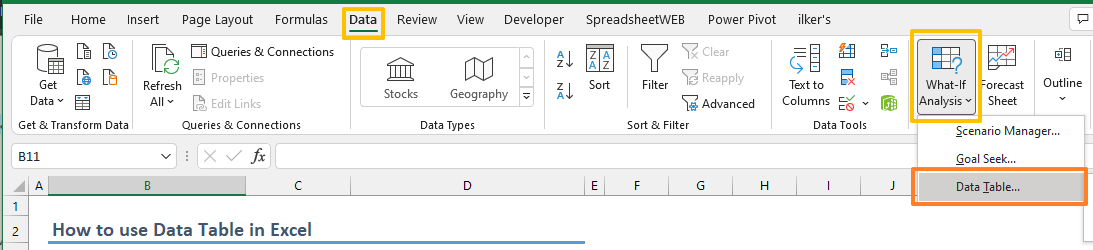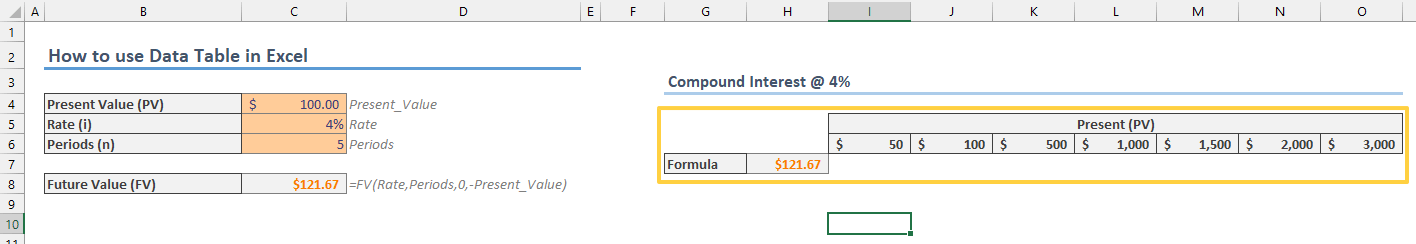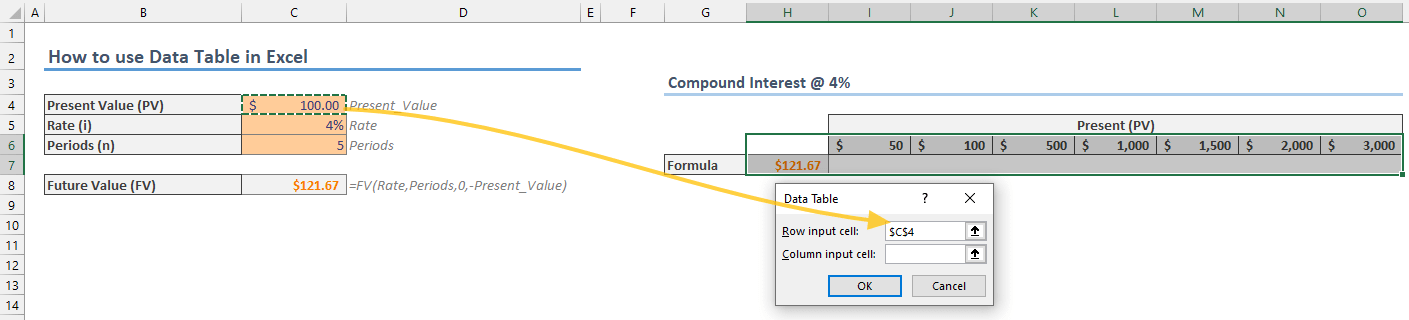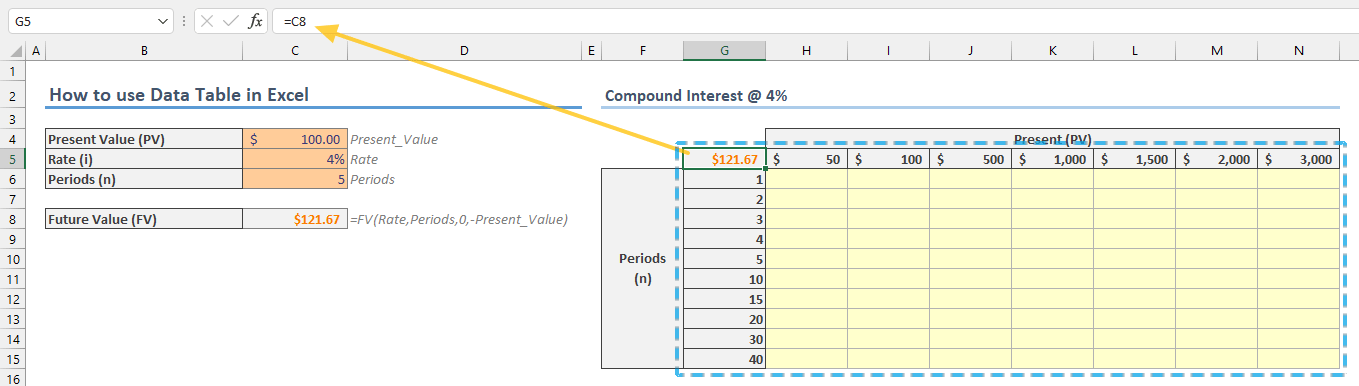A data table can show the results for all possible outcomes at once and saves you from testing against variables individually in your Excel models. In this guide, we’re going to show you how to use Data Table in Excel with one and two variables.

## What is a data table in Excel?

Data Table feature is a what-if analysis tool that allows you see the results of a formula based on up to two variables.

For example, you can see how the capital (Present Value) and period count affect the future value of an investment at 4% rate per year. You can see what the formula returns at the intersection of values. The future value of an \$100 investment at 4% after 5 years is equal to \$121.67.The tool is listed under What-If Analysis button in the Data tab of the Ribbon, along with Scenario Manager and Goal Seek.Note that the Excel Table feature is different than the Data Table.

## How to use data table in Excel?

You can use a data table with one or two variables. Like in the sample image above, the variable values should be listed on a single row and/or column. If you want to test with a single variable, you can use either.

Before populating the variable values, make sure that the input cell(s) are on the same sheet as the table.

### Single variable case

The placement of variables and the formula is important. Our first example is for values in a column.

#### Column Variable

1. Enter the values you want to test into a single-column range (B14:B23). The title is optional.2. Select the cell one row above and one cell to the right of the variable values (C13).
3. Either type in your formula or just give a reference to a cell with formula. In this example, we gave a reference (=C8).4. Select the range that contains both values and the formula (B13:C23).
5. Open the Data Table dialog by following Data > What-If Analysis > Data Table.6. Fill in the Column input cell with the variable cell the formula depends on (C6).
7. Click OK to create and start using the data table.8. Once you click Ok, you will see that the values are populated under the formula.#### Row variable

If you want to test a single variable, you can populate values in a row as well. The trick is to populate the values in a single row and place the formula cell one column to the left and one row below.Once again, select the values and the formula and open Data Table dialog. This time fill the Row input cell with the variable cell affecting the formula (C4).Clicking OK will fill the empty cells with possible results.### Multi variable case

To build a multi variable table, you must have values for each variable in one dimension: One row, one column. The formula cell must be placed where the value row and value column are meant to intersect.You should fill each input in the Data Table dialog.Click OK to see the results from combinations of the two variables.## Remarks

1. The input cells should be in the same worksheet as the data table.
2. Once the table is created, Excel fills the area of results with TABLE formula as an array (see CSE formulas). This function and the array formula are a placeholder for Excel to recognize the area and avoid user interaction.
3. Since the result area contains an array formula, you cannot edit or delete an individual cell. You must delete all results area to make changes.
4. You can change Excel's calculation options to skip Data Tables. If you do not want your data table to be refreshed with each calculation, select Automatic Except for Data Tables option in Formulas > Calculation Options.5. If you enable Automatic Except for Data Tables option, press F9 key to recalculate the worksheet along with the tables.